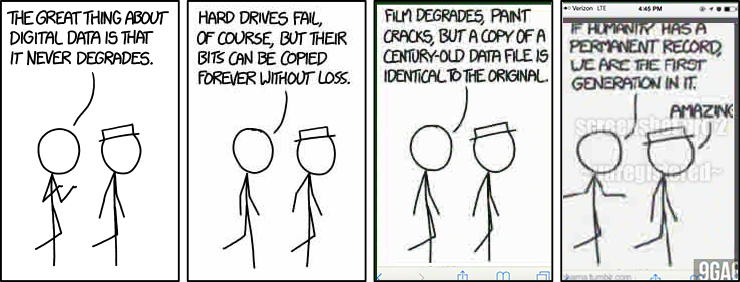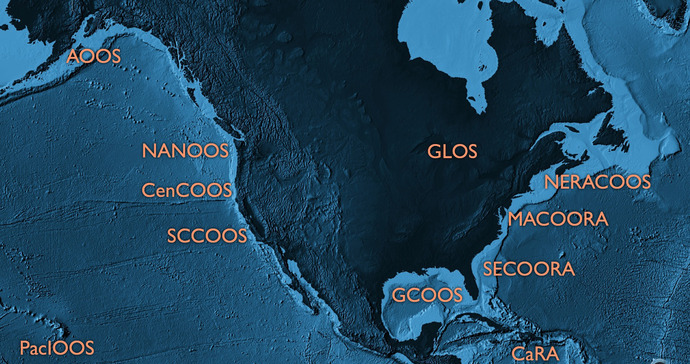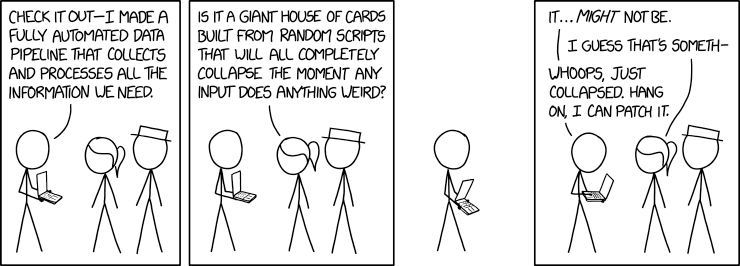```from pandas.plotting import register_matplotlib_converters

register_matplotlib_converters()

import warnings

warnings.filterwarnings("ignore")
```

# Data Access#

## servers, servers everywhere and not a bit to flip## whoami#

`ocefpaf` (Filipe Fernandes)

• Physical Oceanographer

• Data Plumber

• Code Janitor

• CI babysitter

• Amazon-Dash-Button for conda-forge

# My day job: IOOS## Big or small we need data!#

• There are various sources: variety of servers, APIs, and web services. Just to list a few: OPeNDAP, ERDDAP, THREDDS, ftp, http(s), S3, LAS, etc.# Feedback#

As you suffer from my tutorial on Data Access I’d love that you keep the following questions in mind so we can improve the tutorials. Should this tutorial focus on?

• Leveraging metadata for finding data and exploring data?

• Software packages to access, slice, and dice data?

• Data sources?

• None of the above, we don’t need this tutorial!

# Web Services/Type of servers#

Data Type

Web Service

Response

In-situ data
(buoys, stations, etc)

OGC SOS

XML/CSV

Gridded data (models, satellite)

OPeNDAP

Binary

Raster Images

OGC WMS

GeoTIFF/PNG

ERDDAP

Restful API

*

• Your imagination is the limit!

# What are we going to see in this tutorial?#

Browse and access data from:

1. ERDDAP

2. OPeNDAP

3. ~~SOS~~

4. WMS

5. CSW and CKAN*

* There are many examples on CSW in [the IOOS code lab] jupyter-book (https://ioos.github.io/ioos_code_lab/content/intro.html).

# 1) ERDDAP#

## Learning objectives:#

• Explore an ERDDAP server with the python interface (erddapy);

• Find a data for a time/region of interest;

• Download the data with a familiar format and create some plots.

# What is ERDDAP?#

• Flexible outputs: .html table, ESRI .asc and .csv, .csvp, Google Earth .kml, OPeNDAP binary, .mat, .nc, ODV .txt, .tsv, .json, and .xhtml

• RESTful API to access the data

• Standardize dates and time in the results

• Server-side searching and slicing

```from erddapy import ERDDAP

server = "http://erddap.dataexplorer.oceanobservatories.org/erddap"

e = ERDDAP(server=server, protocol="tabledap")
```

## What services are available in the server?#

```import pandas as pd

e.get_search_url(
response="csv",
search_for="all",
)
)
```
```print(
f'We have {len(set(df["tabledap"].dropna()))} '
f'tabledap, {len(set(df["griddap"].dropna()))} '
f'griddap, and {len(set(df["wms"].dropna()))} wms.'
)
```

## Let’s query all the datasets that have the standard_name of sea_water_practical_salinity.#

```url = e.get_categorize_url(
categorize_by="standard_name",
value="sea_water_practical_salinity",
response="csv",
)

dataset_ids = df.loc[~df["tabledap"].isnull(), "Dataset ID"].tolist()

dataset_ids_list = "\n".join(dataset_ids)
print(f"Found {len(dataset_ids)} datasets")
```

## Let us narrow our search to deployments that within a lon/lat/time extent.#

```from ipyleaflet import FullScreenControl, Map, Rectangle

min_lon, max_lon = -72, -69
min_lat, max_lat = 38, 41

rectangle = Rectangle(bounds=((min_lat, min_lon), (max_lat, max_lon)))

m = Map(
center=((min_lat + max_lat) / 2, (min_lon + max_lon) / 2),
zoom=6,
)

```
```m
```
```kw = {
"min_time": "2016-07-10T00:00:00Z",
"max_time": "2017-02-10T00:00:00Z",
"min_lon": min_lon,
"max_lon": max_lon,
"min_lat": min_lat,
"max_lat": max_lat,
"standard_name": "sea_water_practical_salinity",
}
```
```search_url = e.get_search_url(response="csv", **kw)
dataset_ids = search["Dataset ID"].values

dataset_ids_list = "\n".join(dataset_ids)
```
```print(f"Found {len(dataset_ids)} Datasets:\n{dataset_ids_list}")
```
```sal = "sea_water_practical_salinity_profiler_depth_enabled"
temp = "sea_water_temperature_profiler_depth_enabled"

e.dataset_id = dataset_ids

e.variables = [
"z",
"latitude",
"longitude",
sal,
temp,
"time",
]

print(url)
```
```import pandas as pd

df = e.to_pandas(index_col="time (UTC)", parse_dates=True).dropna()

```

Exercise: experiment with the `e.to_xarray()` method. Think about why/where use one or the other?

```import matplotlib.pyplot as plt

subset = df.loc[df["z (m)"] == df["z (m)"].min()]

fig, ax = plt.subplots(figsize=(13, 3.75))
subset[f"{sal} (1e-3)"]["2016"].dropna().plot(ax=ax)
```
```import gsw
import numpy as np

def plot_ts():
fig, ax = plt.subplots(figsize=(5, 5))

s = np.linspace(0, 42, 100)
t = np.linspace(-2, 40, 100)

s, t = np.meshgrid(s, t)
sigma = gsw.sigma0(s, t)

cnt = np.arange(-7, 40, 5)
cs = ax.contour(s, t, sigma, colors="gray", levels=cnt)
ax.clabel(cs, fontsize=9, inline=1, fmt="%2i")

ax.set_xlabel("Salinity [g kg\$^{-1}\$]")
ax.set_ylabel("Temperature [\$^{\circ}\$C]")
ax.scatter(df[f"{sal} (1e-3)"], df[f"{temp} (degree_Celsius)"], s=10, alpha=0.25)

ax.grid(True)
ax.axis([20, 40, 4, 26])
return fig, ax
```
```fig, ax = plot_ts()
```
```responses = ["mat", "json", "ncCF", "ncCFHeader"]

for response in responses:
```

Exercise: explore the web interface for the OOI server URL:

http://erddap.dataexplorer.oceanobservatories.org/erddap/index.html

or the IOOS glider dac:

https://gliders.ioos.us/erddap

and find a dataset of interested, download a format that you are familiar with and plot it (using the web interface or the Python, your choice).

# 2) OPeNDAP#

## Learning objectives:#

• Open model data from a THREDDS server via OPeNDAP with `xarray`;

• Discuss the differences with an `erddapy` request;

• Plot it using `xarray` interface.

```import cf_xarray
import xarray as xr

url = (
"http://tds.marine.rutgers.edu/thredds/dodsC/roms/doppio/2017_da/avg/Averages_Best"
)
ds = xr.open_dataset(url)
ds.cf
```
```variable = "sea_water_potential_temperature"
time = "2022-08-10"
surface = -1

selection = ds.cf[variable].sel(time="2022-08-10").isel(s_rho=surface)
```
```import cartopy.crs as ccrs
import matplotlib.pyplot as plt

fig, ax = plt.subplots(
figsize=(6, 6),
subplot_kw={"projection": ccrs.PlateCarree()},
)

selection.plot(
ax=ax,
x="lon_rho",
y="lat_rho",
)

ax.coastlines()
```

# 3) ~~SOS~~#

## Learning objectives:#

• Use searvey to obtain CO-OPS data

```import shapely
from searvey import coops

secoora = shapely.geometry.box(-87.4, 24.25, -74.7, 36.7)
df = coops.coops_stations_within_region(secoora)
df
```
```df.loc[df["name"] == "Duck"]
```
```from datetime import datetime, timedelta

from searvey.coops import COOPS_Station

station = COOPS_Station("Duck")

ds = station.product(
"water_level",
start_date=datetime.today() - timedelta(15),
end_date=datetime.today(),
)

ds["v"].plot()
```

# 4) WMS#

## Learning objectives:#

• Add a WMS layer to an interactive map. (“Hurricane viz widget.”)

```from ipyleaflet import FullScreenControl, Map, WMSLayer, basemaps
from ipywidgets import SelectionSlider
from traitlets import Unicode

time_options = [
"13:00",
"13:30",
"14:00",
"14:30",
"15:00",
"15:30",
"16:00",
"16:30",
"17:00",
"17:30",
"18:00",
"18:30",
]

slider = SelectionSlider(description="Time:", options=time_options)

def update_wms(change):
time_wms.time = "2020-07-25T{}".format(slider.value)

slider.observe(update_wms, "value")

class TimeWMSLayer(WMSLayer):
time = Unicode("").tag(sync=True, o=True)
```
```time_wms = TimeWMSLayer(
time="2020-07-25T13:00:00Z",
format="image/png",
transparent=True,
)
m = Map(basemap=basemaps.CartoDB.Positron, center=(30, -88), zoom=5)
```
```m
```
```slider
```

# 5) Catalog Service Web (CSW)#

## Is there a canonical source for data?#Well, kind of… The closet thing is are data catalogs like the IOOS CSW catalog or pangeo-forge.

# Catalog Service for the Web (CSW)#

• A single source to find endpoints

• Nice python interface:
`owslib.csw.CatalogueServiceWeb`

`owslib.fes`# Where to find data?#

Curated list of ERDDAP servers: https://github.com/IrishMarineInstitute/awesome-erddap

Environmental Data Service (EDS) model viewer: https://eds.ioos.us

Exploring THREDDS servers: https://unidata.github.io/siphon/latest

# Standards!#```import cftime
import nc_time_axis
from netCDF4 import Dataset

url = "http://goosbrasil.org:8080/pirata/B19s34w.nc"
nc = Dataset(url)

temp = nc["temperature"][:]
times = nc["time"]
temp[temp <= -9999] = np.NaN
t = cftime.num2date(times[:], times.units, calendar=times.calendar)
```
```mask = (t >= datetime(2008, 1, 1)) & (t <= datetime(2008, 12, 31))
```
```fig, ax = plt.subplots()
```import xarray as xr
```temp.sel(depth_t=1.0, time="2008").plot()